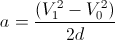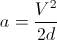Accelerate based on distance

Here is how to specify acceleration or deceleration based on distance:

First you need to know the initial velocity, the final velocity, and the desired distance.

Use the equationMake a user program to calculate the acceleration and use it in a move command.

In the example below, the initial velocity for the acceleration phase is zero, and the final velocity for the deceleration phase is zero, so the equation for both phases is simplyDelta Computer Systems Website